# Optical Flow

### 1. Background

Optical flow is the approximated motion vector at each pixel location. It can tell us about the relative distances of objects, as closer moving objects will have more apparent motion than moving objects that are further away, given equal speed. The explanation on this page follows the treatment in .

Optical flow is based on the assumptions that objects in the image at time t will generally still be in the image at time t+1, only they will be displaced. This is represented by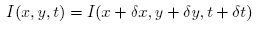This leads via Taylor series expansion to the 2D motion constraint equation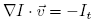This one equation has two unknowns and so does not have a unique solution. This is the mathematical consequence of the aperture problem. The aperture problem is that there is not enough information in a small area to uniquely determine motion (this is what causes the barber pole illusion).

To add an additional constraint, Horn and Schunck  use a global regularization calculation. They assume that images consist of objects undergoing rigid motion, and so over relatively large areas the optical flow will be smooth. They then minimize the square of the magnitude of the gradient of optical flow using the equationIn contrast, Lucas and Kanade  use a local least squares calculation to provide the constraint by minimizing in the neighborhood surrounding the pixel, represented in equation form by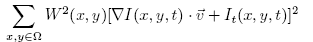### 2. Implementation

Given the image sequence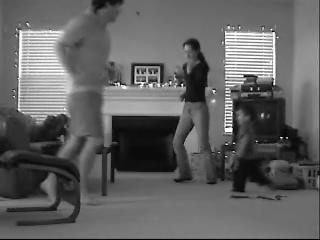All partial derivatives were calculated as was outlined in . The partial spatial derivative in the x direction and the partial spatial derivative in the y direction are shown here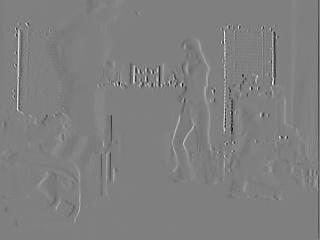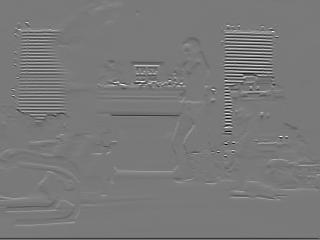and the partial derivative in time direction is shown hereUsing Horn and Schunck  the calculated optical flow for the example images isand using Lucas and Kanade  it is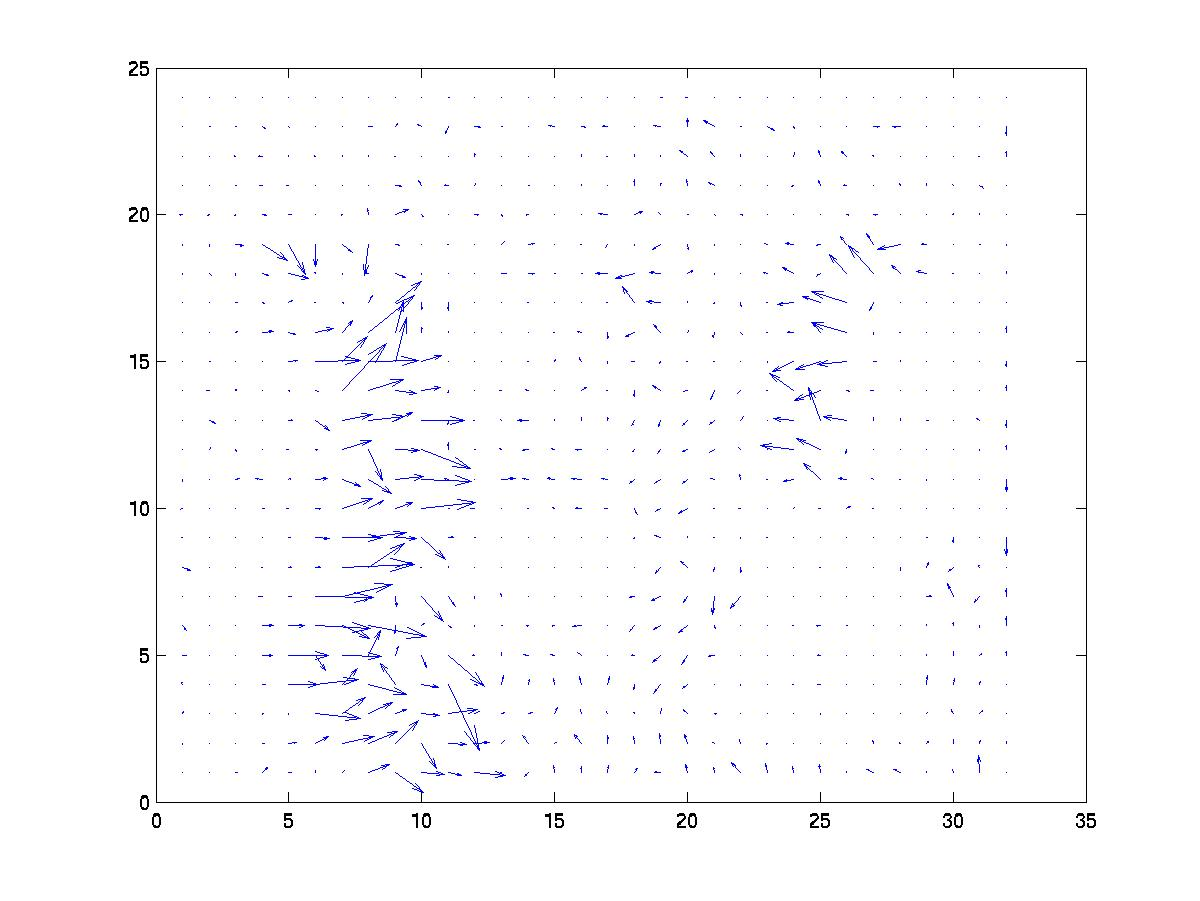Both methods were able to identify me running from the left to the right. My wife's movement was more subtle and that was represented in the flow, however the movement of my son was not represented due to his partial spatial derivatives being lost in the clutter of the television set.

It did less well with movement towards the camera. Given the framesUsing Horn and Schunck  the calculated optical flow for the example images isand using Lucas and Kanade  it isHere is a video (1.4 MB) of the optical flow calculated as in  from the movie in the discovering objects section of the project.

### References

 J.L. Barron and N.A. Thacker, "Tutorial: Computing 2D and 3D Optical Flow", Tina Memo No. 2004-012, 2005.
 B.K.P. Horn and B.G. Schunck, "Determining Optical Flow", Artificial Intelligence, 17, 1981, 185-204.
 B.D. Lucas and T. Kanade, "An Iterative Image Registration Technique with an Application to Stereo Vision", DARPA Image Understanding Workshop, 1981, 121-130.

I wrote a book called The Curiosity Cycle: Preparing Your Child for the Ongoing Technological Explosion.